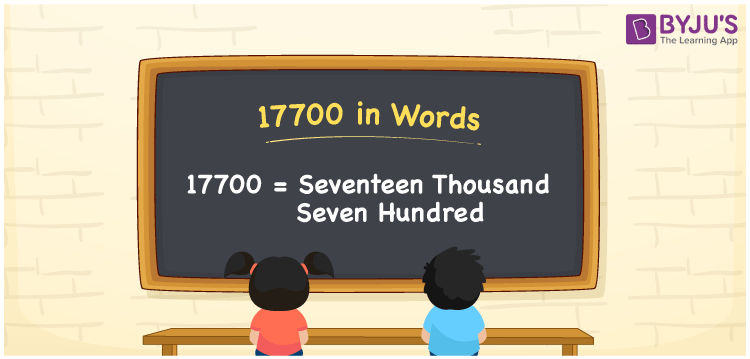# 17700 in words

17700 in words is written as Seventeen Thousand Seven Hundred. In 17700, 1’s position is in ten thousands place, first 7’s position is in thousands place, second 7’s position is in hundred’s place. The article on Place Value gives more information. The number 17700 is used in expressions that relate to money, distance, length, population and many more. For example, “The minimum price for a smart phone starts from Rupees Seventeen Thousand Seven Hundred onwards.” Another example is “In the number 17700, seven is repeated twice”.

 17700 in words Seventeen Thousand Seven Hundred Seventeen Thousand Seven Hundred in Numbers 17700

## 17700 in English Words## How to Write 17700 in Words?

We can convert 17700 to words using a place value chart. The number 17700 has 5 digits, so let’s make a chart that shows the place value up to 5 digits.

 Ten thousand Thousands Hundreds Tens Ones 1 7 7 0 0

Thus, we can write the expanded form as:

1 × Ten thousand + 7 × Thousand + 7 × Hundred + 0 × Ten + 0 × One

= 1 × 10000 + 7 × 1000 + 7 × 100 + 0 × 10 + 0 × 1

= 17700

= Seventeen Thousand Seven Hundred.

17700 is the natural number that is succeeded by 17699 and preceded by 17701.

17700 in words – Seventeen Thousand Seven Hundred.

Is 17700 an odd number? – No.

Is 17700 an even number? – Yes.

Is 17700 a perfect square number? – No.

Is 17700 a perfect cube number? – No.

Is 17700 a prime number? – No.

Is 17700 a composite number? – Yes.

## Solved Example

1. Write the number 17700 in expanded form

Solution: 1 x 10000 + 7 x 1000 + 7 x 100 + 0 x 10 + 0 x 1

Or Just 1 x 10000 + 7 x 1000 + 7 x 100

We can write 17700 = 10000 + 7000 + 700 + 0 + 0

= 1 x 10000 + 7 x 1000 + 7 x 100 + 0 x1 0 + 0 x 1

## Frequently Asked Questions on 17700 in words

Q1

### How to write 17700 in words?

17700 in words is written as Seventeen Thousand Seven Hundred.
Q2

### State True or False. 17700 is divisible by 7?

False. 17700 is not divisible by 7.
Q3

### Is 17700 divisible by 10?

Yes. 17700 is divisible by 10.